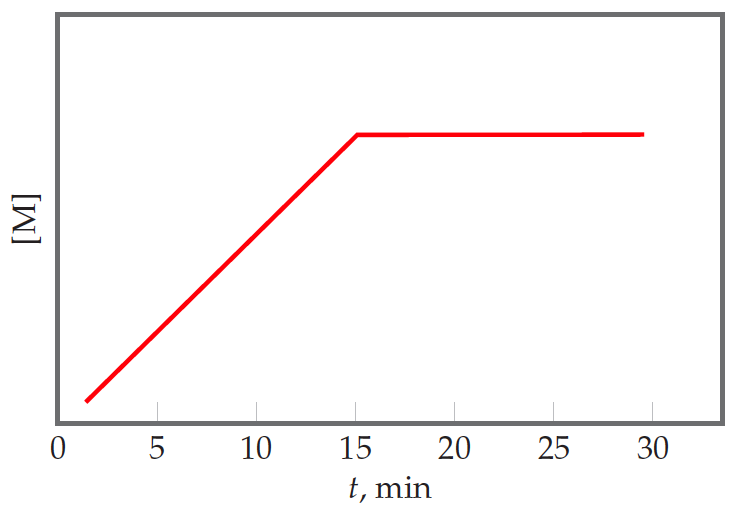# Problem: Suppose that for the reaction K + L → M, you monitor the production of M over time, and then plot the followinggraph from your data:Is the reaction occurring at a constant rate from t = 0 to t = 15 min?

###### FREE Expert Solution
83% (197 ratings)
###### Problem Details

Suppose that for the reaction K + L → M, you monitor the production of M over time, and then plot the following